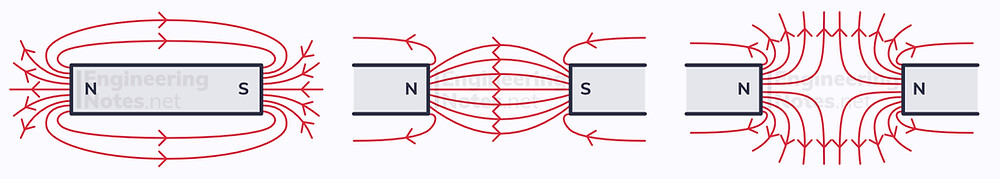#### Notes by Category University Engineering

Electronics*
Mathematics*
Mechanics & Stress Analysis*
Rate these notesNot a fanNot so goodGoodVery goodBrillRate these notes
• A-Level Physics

# Magnetism & Electromagnetism

A magnetic field is a region in which a magnetic material experiences a force, and is caused by a moving charge or a permanent magnet.Just like gravitational and electric fields, magnetic fields are represented by field lines - except instead of talking about positive and negative end, we talk about north and south poles. The closer the field lines, the stronger the magnetic field. Identical to charges, like poles repel and opposite poles attract.

The diagram above shows the field patterns from permanent bar magnets, but magnetic fields arise all the time from current-carrying wires:• For a straight current-carrying wire, the field lines are arranged in concentric circles in a plane perpendicular to the wire and direction of current. This is found using the right-hand grip rule.

• A flat current-carrying coil produces the same pattern, but all the way around the coil, so the field is three-dimensional.

• A solenoid produces this pattern on an elongated scale.

## Magnetic Flux Density

A current that is perpendicular to a uniform magnetic field will induce a force. The direction of this force can be established with Fleming's left-hand rule:The magnitude of the force causing the motion is proportional to the magnetic flux density, B, of the magnetic field. This is defined as the force on one meter of wire carrying a current of 1 amp, perpendicular to the magnetic field.

F = BIL sinθ Force = flux density x current x length of wire x sin(angle with magnetic field)
• If the wire is perpendicular, the equation is just F = BIL, as sin(90°) = 1

• If the wire is parallel, there is no force, as sin(0°) = 0

This relationship can be investigated with a known length of wire on a scale, running perpendicular through a uniform magnetic field. When a known current passes through the wire, the scale will read a change in mass. This, multiplied by g, is equivalent to the force exerted on the wire.